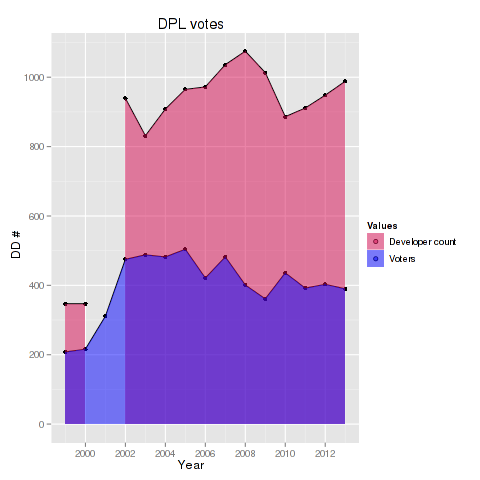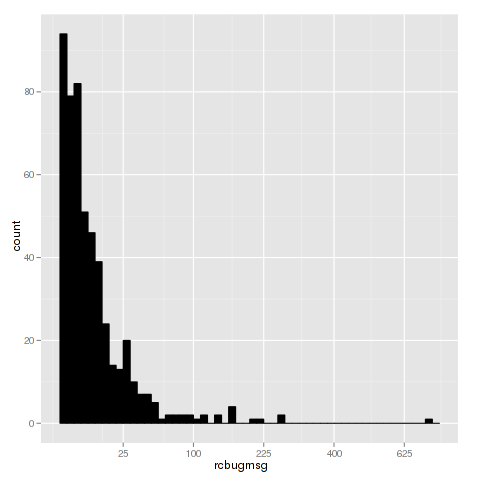# Ulrich Dangel

24 April 2013

Axel ‘XTaran’ Beckert recently asked in `#debian-devel` for a visualization of the Debian Project Leader Election results. Unfortunately there seems to be none, except the table in the mail. As I am currently trying to use `ggplot2` for more things, I thought I would give it a try and convert the data into a csv file and process the data via `R`.

For converting the original data i used `vim` to do a little text processing and created a csv file. By running the following code we can create a nice looking simple graphic:

``````library("ggplot2")

p <- ggplot(votes2, aes(Year, value, group=variable, fill=variable)) + ylab('DD #')
p <- p + geom_point() + geom_line() + geom_area(position='identity')
p <- p + scale_fill_manual("Values", values=c(alpha('#d70a53', 0.5),
alpha("blue", 0.5)), breaks=c("DDs", "unique"), labels=c("Developer count", "Voters"))

print(p)
``````I also created a little script for automatically processing the csv file and creating a similar plot. Feel free to fork/clone extend this script.

21 April 2013

# Analyzing rc bug messages

Michael Stapelberg recently posted a blog post about looking into the number of Debian Developers actively working on RC bugs for the upcoming wheezy release.

In this blog post I analyze the data shared by Michael and provide the `R` commands used to generate the plots & findings. If you are interested into looking into the data yourself, but don’t like `R`, I suggest using ipython notebook + numpy instead.

## Analysis

After parsing the data file we typically want to get an understanding of the data, by using `summary(bugs)` we get the `minimum(1)`, `median(5)`, `mean(15.4)`, `max(716)` and quantiles of the data. This shows that the number of messages is wide-spread and a few people contribute a lot. To visualize the dispersion of the data we can create a box plot showing the range of messages:As the first and third quantile are close together we can assume that the majority of the work is done by a few, especially since the second quantile is 5. This is supported by the histogram below, where the x axis is the number of recorded messages and y is the number of developers.## Top 10 contributors

The TOP 10 contributors, according to the dataset, are:

1. Lucas Nussbaum - 716 messages
2. Gregor Herrmann - 270 messages
3. Jakub Wilk - 270 messages
4. Andreas Beckmann - 225 messages
5. Julien Cristau - 205 messages
6. Cyril Brulebois - 169 messages
7. Moritz Muehlenhoff - 162 messages
8. Michael Biebl - 159 messages
9. Salvatore Bonaccorso - 158 messages
10. Christoph Egger - 142 messages

## r commands

These are the commands used to generate the plots and information in this plot:

``````bugs <- read.csv("by-msg.csv")
summary(bugs)
boxplot(bugs\$rcbugmsg, log='y', range=0, ylab="# bugs")
quantile(bugs\$rcbugmsg)
0%  25%  50%  75% 100%
1    2    5   12  716

# create histogram
llibrary('ggplot2')
ggplot(bugs, aes(x=rcbugmsg)) + geom_histogram(binwidth=.5, colour="black", fill="black") + scale_x_sqrt()

top10 <- tail(bugs[order(bugs\$rcbugmsg),], 10)
top10
``````